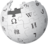# Theory of Relativity

From Halopedia, the Halo wikiThere is more information available on this subject at Theory of Relativity on the English Wikipedia.

The Theory of Relativity , or simply relativity, refers specifically to two theories of Albert Einstein: special relativity and general relativity. However, "relativity" can also refer to Galilean relativity.

The term "Theory of Relativity" was coined by Max Planck in 1908 to emphasize how special relativity (and later, general relativity) uses the principle of relativity.

## Special relativity

Special relativity is a theory of the structure of spacetime. It was introduced in Albert Einstein's 1905 paper "On the Electrodynamics of Moving Bodies". Special relativity is based on two postulates which are contradictory in classical mechanics:

• The laws of physics are the same for all observers in uniform motion relative to one another (Galileo's principle of relativity),
• The speed of light in a vacuum is the same for all observers, regardless of their relative motion or of the motion of the source of the light.

The resultant theory has many surprising consequences. Some of these are:

• Time dilation: Moving clocks are measured to tick more slowly than an observer's "stationary" clock.
• Length contraction: Objects are measured to be shortened in the direction that they are moving with respect to the observer.
• Relativity of simultaneity: two events that appear simultaneous to an observer A will not be simultaneous to an observer B if B is moving with respect to A.
• Mass-energy equivalence: E = mc², energy and mass are equivalent and transmutable.

The defining feature of special relativity is the replacement of the Galilean transformations of classical mechanics by the Lorentz transformations. (See Maxwell's Equations of electromagnetism and introduction to special relativity).

## General Relativity

General relativity is a theory of gravitation developed by Einstein in the years 1907–1915. The development of general relativity began with the equivalence principle, under which the states of accelerated motion and being at rest in a gravitational field (for example when standing on the surface of the Earth) are physically identical. The upshot of this is that free fall is inertial motion: In other words an object in free fall is falling because that is how objects move when there is no force being exerted on them, instead of this being due to the force of gravity as is the case in classical mechanics. This is incompatible with classical mechanics and special relativity because in those theories inertial moving objects cannot accelerate with respect to each other, but objects in free fall do so. To resolve this difficulty Einstein first proposed that spacetime is curved. In 1915, he devised the Einstein field equations which relate the curvature of spacetime with the mass, energy, and momentum within it.

Some of the consequences of general relativity are:

• Time goes more slowly at lower gravitational potentials. This is called gravitational time dilation.
• Orbits precess in a way unexpected in Newton's theory of gravity. (This has been observed in the orbit of Mercury and in binary pulsars).
• Even rays of light (which are weightless) bend in the presence of a gravitational field.
• The Universe is expanding, and the far parts of it are moving away from us faster than the speed of light. This does not contradict the theory of special relativity, since it is space itself that is expanding.
• Frame-dragging, in which a rotating mass "drags along" the space time around it.

Technically, general relativity is a metric theory of gravitation whose defining feature is its use of the Einstein field equations. The solutions of the field equations are metric tensors which define the topology of the spacetime and how objects move inertial.

## Relativity and the Slipstream

Because the Theory of Relativity prohibits travel at speeds faster than light, or at the very least imposes extreme effects upon objects traveling at those speeds, both the UNSC and the Covenant instead use a different medium to travel at faster-than-light speeds, the slipstream. The laws of relativity are different in the slipstream, allowing far easier and faster travel than the regular universe. Unfortunately, this can sometimes have detrimental effects on those using it.

Due to the hyperdimensional nature of slipspace, the space-curving effect exhibited by mass is magnified by several orders of magnitude. Effectively, this means that gravity is stronger in slipspace.